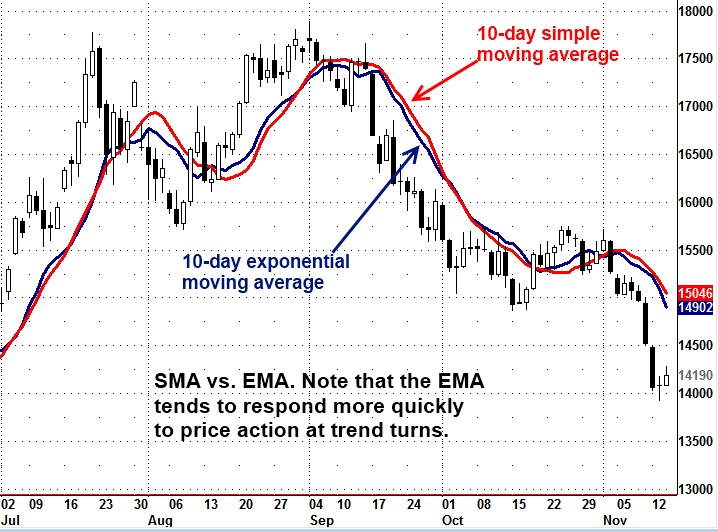Background: Perhaps the simplest to understand and most widely used technical indicator is a moving average, which traders have used for many years to smooth out erratic short-term price fluctuations to reveal existing trends or situations where a trend may be ready to begin or about to reverse.

The close is often the one price point used for a given period, but a moving average may also be based on the open, high or low or some combination of price points. There are three main types of moving averages:

Simple Moving Average (SMA) Just add the prices for a specified period of time and divide by the number of prices in that period to get an average. Each price is given an equal weight. As each new price becomes available, the oldest price is dropped from the calculation.

Weighted Moving Average More weight is given to the latest price, which is regarded as more important than older prices. If you are calculating a three-day weighted moving average, for example, the latest price might be multiplied by 3, yesterday’s price by 2 and the oldest price three days ago by 1. The sum of these figures is divided by the sum of the weighting factors – 6 in this example. This makes the weighted moving average more responsive to current price changes.

Exponential Moving Average (EMA) An exponential moving average (EMA) is another form of a weighted moving average that gives more importance to the most recent prices. Instead of dropping off the oldest prices in the calculation, however, all past prices are factored into the current average. The current EMA is calculated by subtracting yesterday’s EMA from today’s price, multiplying the result by a constant and then adding this result to yesterday’s EMA to get today’s EMA. An EMA incorporates all past price data and generally produces a smoother line than other forms of moving averages, which can be an important factor in choppy market conditions.

Purpose: Moving averages have several uses: (1) Reveal trends by smoothing out data when market “noise” produces erratic price patterns, (2) identify points where trends may be ready to begin or end, (3) indicate shifts in market momentum based on the performance of price vs. a moving average or one moving average vs. another.

Basic signals: The simplest signal involves only price and one moving average. When the price is above the moving average, be long; when price is below the moving average, be short.

Moving averages are often used in crossover trading systems. A buy signal occurs when a short-term or intermediate-term moving average crosses from below to above a longer-term moving average. Conversely, a sell signal is issued when the short-term or intermediate-term average crosses from above to below the longer-term average.

Because the moving average changes constantly with each new price data input, many traders test different time frames before they come up with a series of moving averages that are optimal for a particular market. The shorter a moving average, the more sensitive it will be to price movements. Traders will have to adjust the length of a moving average and how to use its signals to suit their own trading style.

Some traders use combinations of three moving averages of different lengths, such as 5-day, 10-day and 20-day moving averages or 4-, 9- and 18-period moving averages, taking crossovers of the shorter and medium-term moving average above/below the longer moving average for trade entry and then perhaps use the shorter moving average as a stop point. Still others – primarily those trading stocks – use longer-term moving average lines, such as 50-day, 100-day or 200-day as another point of support or resistance.

Pros/cons: Easy to understand and implement, especially since various types of moving averages are included in analytical software packages so traders do not have to calculate the averages by hand. They give a mechanical trading system a precise price to take action, reducing subjectivity.

One negative is that moving averages are a lagging indicator – that is, they are based on past price data and their movements usually trail current price action.